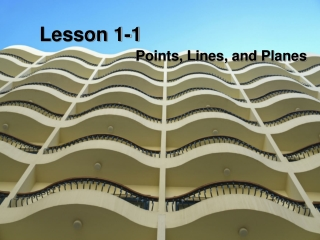DownloadDownload PresentationLesson 1-1

# Lesson 1-1

Télécharger la présentation## Lesson 1-1

- - - - - - - - - - - - - - - - - - - - - - - - - - - E N D - - - - - - - - - - - - - - - - - - - - - - - - - - -
##### Presentation Transcript

1. Lesson 1-1 Points, Lines, and Planes

2. Ohio Content Standards:

3. Ohio Content Standards: • Formally define geometric figures.

4. Ohio Content Standards: • Formally define geometric figures. • Recognize and explain the necessity for certain terms to remain undefined, such as point, line and plane

5. Vocabulary:

6. Vocabulary: • Point:

7. Vocabulary: • Point: simply a location

8. Vocabulary: • Line:

9. Vocabulary: • Line: made up of points and has no thickness or width

10. Vocabulary: • Collinear:

11. Vocabulary: • Collinear: points on the same line

12. Vocabulary: • Plane:

13. Vocabulary: • Plane: flat surface made up of points

14. Vocabulary: • Coplanar:

15. Vocabulary: • Coplanar: Points that lie on the same plane

16. Use the figure to name each of the following:

17. Use the figure to name each of the following: • a line containing point K J K M L

18. Use the figure to name each of the following: • a line containing point K • a plane containing point L J K M L

19. Name the geometric shape modeled by each object.

20. Name the geometric shape modeled by each object. • the long hand on a clock

21. Name the geometric shape modeled by each object. • the long hand on a clock • a 10 X 12 patio

22. Name the geometric shape modeled by each object. • the long hand on a clock • a 10 X 12 patio • the location where the corner of a driveway meets the road

23. Vocabulary:

24. Vocabulary: • Undefined terms:

25. Vocabulary: • Undefined terms: terms only explained using examples and descriptions

26. Draw and label a figure for each relationship.

27. Draw and label a figure for each relationship. • plane R contains lines AB and DE, which intersect at point P. Add point C on plane R so that it is not collinear with AB or DE.

28. Draw and label a figure for each relationship. • plane R contains lines AB and DE, which intersect at point P. Add point C on plane R so that it is not collinear with AB or DE. • QR on a coordinate plane contains Q(-2, 4) and R(4, -4). Add point T so that T is collinear with these points.

29. A C B S D

30. A C B • How many planes appear in this figure? S D

31. A C B • How many planes appear in this figure? • Name three points that are collinear. S D

32. A C B • How many planes appear in this figure? • Name three points that are collinear. • Are points A, B, C, and D coplanar? Explain. S D

33. A C B • How many planes appear in this figure? • Name three points that are collinear. • Are points A, B, C, and D coplanar? Explain. • At what point do DB and CA intersect? S D

34. Assignment: Pgs. 9 - 11 14 - 46 evens